### IMO Shortlist 1999 problem C1

Kvaliteta:
Avg: 0,0
Težina:
Avg: 6,0
Let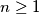$n \geq 1$ be an integer. A path from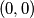$(0,0)$ to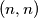$(n,n)$ in the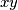$xy$ plane is a chain of consecutive unit moves either to the right (move denoted by$E$) or upwards (move denoted by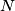$N$), all the moves being made inside the half-plane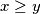$x \geq y$. A step in a path is the occurence of two consecutive moves of the form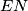$EN$. Show that the number of paths from$(0,0)$ to$(n,n)$ that contain exactly$s$ steps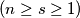$(n \geq s \geq 1)$ is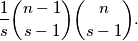Izvor: Međunarodna matematička olimpijada, shortlist 1999